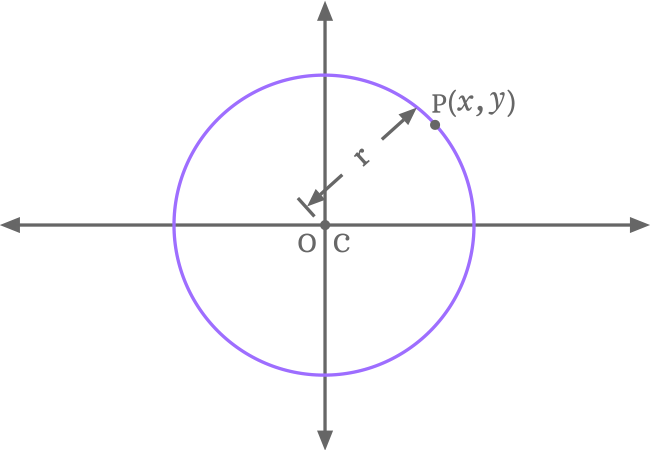# Equation of a circle centered at the origin

## Equation

$x^2+y^2 \,=\, r^2$

### Introduction

A circle without touching any axis of the two dimensional cartesian coordinate system is a standard form a circle and its circle is called the equation of a circle in standard form.Let $C$ represents the center (or centre) of a circle, $P$ represents a point on the circumference of the circle and $r$ represents the radius of circle.

Let $a$ and $b$ be $x$ and $y$ coordinates of center (or centre) and the center in coordinate form is written as $C(a, b)$. Similarly, $x$ and $y$ be the horizontal and vertical coordinates of point $P$ and it is written as $P(x, y)$ in coordinate form.

The equation of a circle in standard form is written in mathematics as follows.

$x^2+y^2 \,=\, r^2$

#### Proof

Learn how to derive the equation of a circle in general form when the circle does not touch both axes.

Latest Math Problems

A best free mathematics education website for students, teachers and researchers.

###### Maths Topics

Learn each topic of the mathematics easily with understandable proofs and visual animation graphics.

###### Maths Problems

Learn how to solve the maths problems in different methods with understandable steps.

Learn solutions

###### Subscribe us

You can get the latest updates from us by following to our official page of Math Doubts in one of your favourite social media sites.